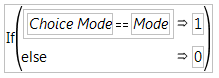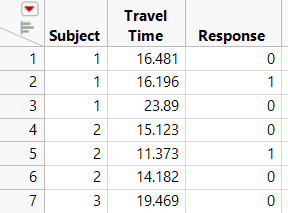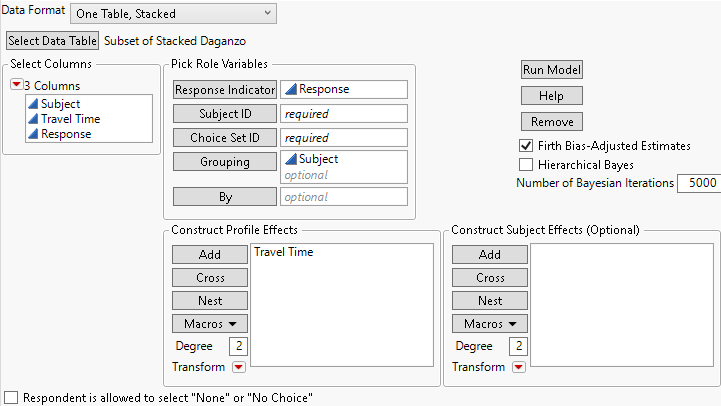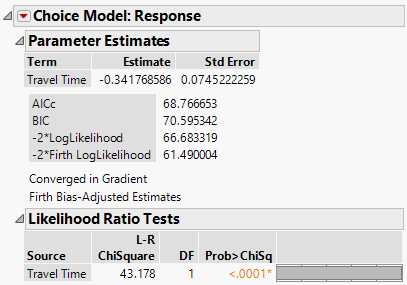Consumer Research > Choice Models > Additional Examples > Example of Transforming Data to One Analysis Table
Publication date: 05/24/2021

## Example of Transforming Data to One Analysis Table

Rather than creating two or three tables, it can be more practical to transform the data so that only one table is used. For the one-table format, the subject effect is added as in the previous example. A response indicator column is added instead of using three different columns for the choice sets (Choice 1, Choice 2, Choice 3). Transform data for use with the one-table scenario:

1. Create or open Stacked Daganzo.jmp from the Stack the Data steps shown in Example of Transforming Data to Two Analysis Tables.

2. Select Cols > New Columns.

3. Type Response as the Column Name.

4. Click Column Properties and select Formula.

5. Select Conditional in the functions list and then select If.

6. Select the column Choice Mode for the expression (expr).

7. Enter “=” and select Mode.

8. Type 1 for the Then Clause and 0 for the Else Clause.

9. Click OK in the Formula Editor window. Click OK in the New Column window.

The completed formula should look like Figure 4.52.

Figure 4.52 Formula for Response Indicator for Stacked Daganzo Data10. Select the Subject, Travel Time, and Response columns and then select Tables > Subset.

11. Select All Rows and Selected Columns and click OK.

A partial listing of the new data table is shown in Figure 4.53.

Figure 4.53 Partial Table of Stacked Daganzo Data Subset12. Select Analyze > Consumer Research > Choice to open the launch window and specify the model as shown in Figure 4.54.

Figure 4.54 Choice Dialog Box for Subset of Stacked Daganzo Data for One-Table Analysis13. Click Run Model.

Figure 4.55 Parameter Estimate for Travel Time of Daganzo Data from One-Table AnalysisNotice that the result is identical to that obtained for the two-table model, shown earlier in Figure 4.51.

This chapter illustrates the use of the Choice Modeling platform with simple examples. This platform can also be used for more complex models, such as those involving more complicated transformations and interaction terms.